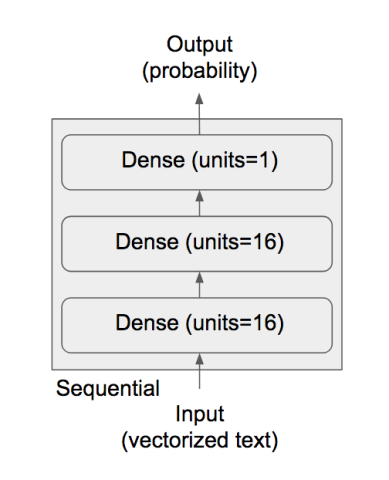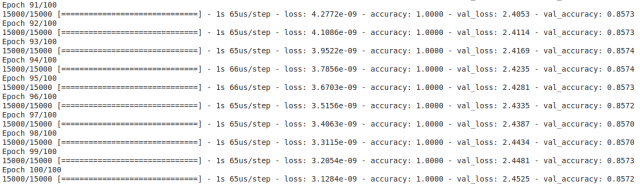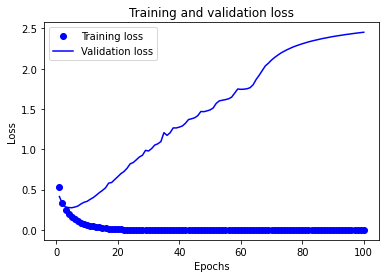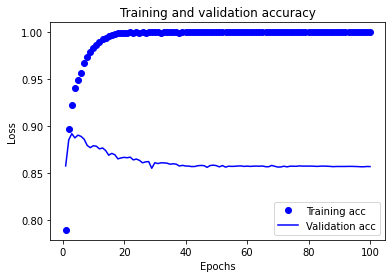# Keras 教學 - 訓練 IMDB Reviews CNN 網路模型

## 關於 IMDB Dataset 資料集

IMDB Dataset 是來自 Internet 電影數據庫 50,000 條評論文字。他們分為 25,000 條訓練數據和 25,000 條測試數據，每組皆包含包括 50％ 的負面評論和 50％ 的正面評論。

```from keras.datasets import imdb
(train_data, train_labels), (test_data, test_labels) = imdb.load_data(num_words=10000)```

num_words=10000 表示我們只採用前 10000 個常出現的字詞，此外在 label 中 0 表示負評 1 表示正評。

`max([max(sequence) for sequence in train_data])`

```word_index = imdb.get_word_index()
reverse_word_index = dict([(value, key) for (key, value) in word_index.items()])
decoded_review = ' '.join([reverse_word_index.get(i - 3, '?') for i in train_data])

# show
decoded_review```

## IMDB Reviews 資料前處理

```import numpy as np

def vectorize_sequences(sequences, dimension=10000):
# Create an all-zero matrix of shape (len(sequences), dimension)
results = np.zeros((len(sequences), dimension))
for i, sequence in enumerate(sequences):
results[i, sequence] = 1.  # set specific indices of results[i] to 1s
return results

# Our vectorized training data
x_train = vectorize_sequences(train_data)
# Our vectorized test data
x_test = vectorize_sequences(test_data)```

```# Our vectorized labels
y_train = np.asarray(train_labels).astype('float32')
y_test = np.asarray(test_labels).astype('float32')```

## 建立 CNN 網路架構• 訓練 Dataset：25,000
• 測試 Dataset：25,000
• 優化器：RMSprop
• 損失函數：二元分類
• Batch Size：512
• Epochs：100
```from keras import models
from keras import layers

model = models.Sequential()

```model.compile(optimizer='rmsprop',
loss='binary_crossentropy',
metrics=['accuracy'])```

## 訓練 CNN 模型

```x_val = x_train[:10000]
partial_x_train = x_train[10000:]

y_val = y_train[:10000]
partial_y_train = y_train[10000:]```

```history = model.fit(partial_x_train,
partial_y_train,
epochs=100,
batch_size=512,
validation_data=(x_val, y_val))``````history_dict = history.history
history_dict.keys()```

## 訓練結果圖表分析

```#@title
import matplotlib.pyplot as plt

acc = history.history['accuracy']
val_acc = history.history['val_accuracy']
loss = history.history['loss']
val_loss = history.history['val_loss']

epochs = range(1, len(acc) + 1)

# "bo" is for "blue dot"
plt.plot(epochs, loss, 'bo', label='Training loss')
# b is for "solid blue line"
plt.plot(epochs, val_loss, 'b', label='Validation loss')
plt.title('Training and validation loss')
plt.xlabel('Epochs')
plt.ylabel('Loss')
plt.legend()

plt.show()``````plt.clf()   # clear figure
acc_values = history_dict['accuracy']
val_acc_values = history_dict['val_accuracy']

plt.plot(epochs, acc, 'bo', label='Training acc')
plt.plot(epochs, val_acc, 'b', label='Validation acc')
plt.title('Training and validation accuracy')
plt.xlabel('Epochs')
plt.ylabel('Loss')
plt.legend()

plt.show()```